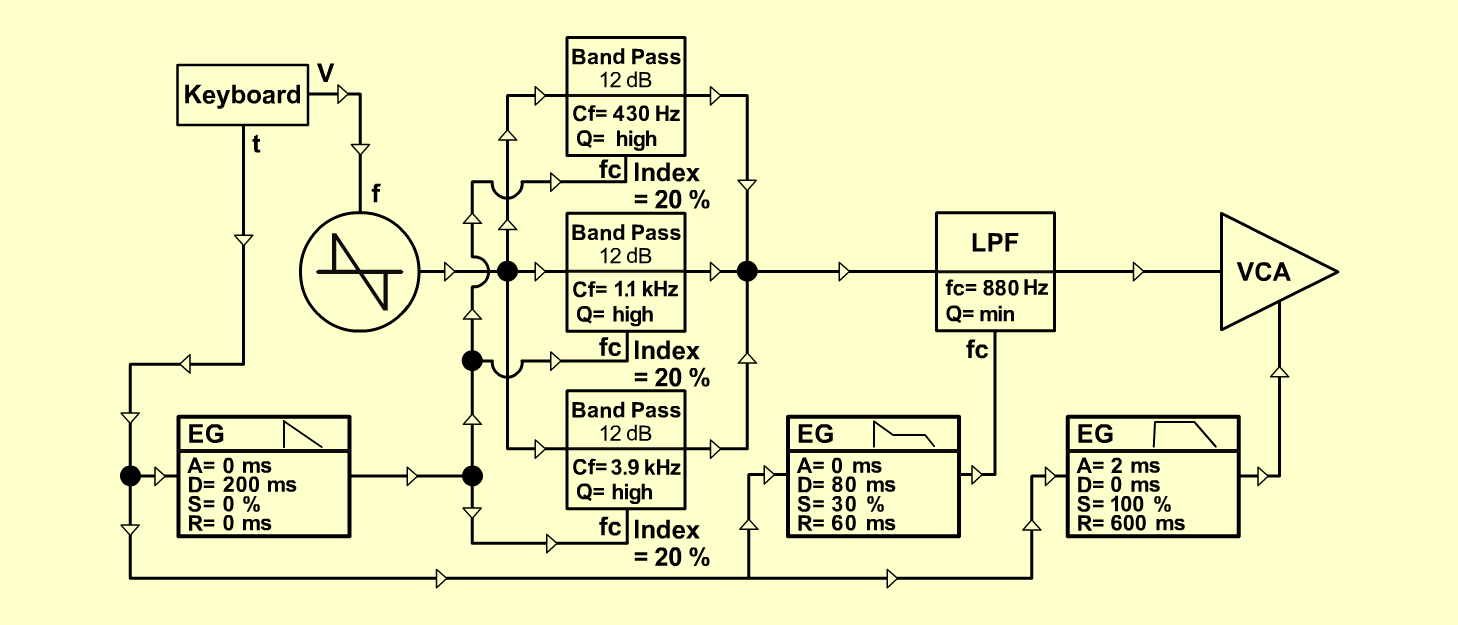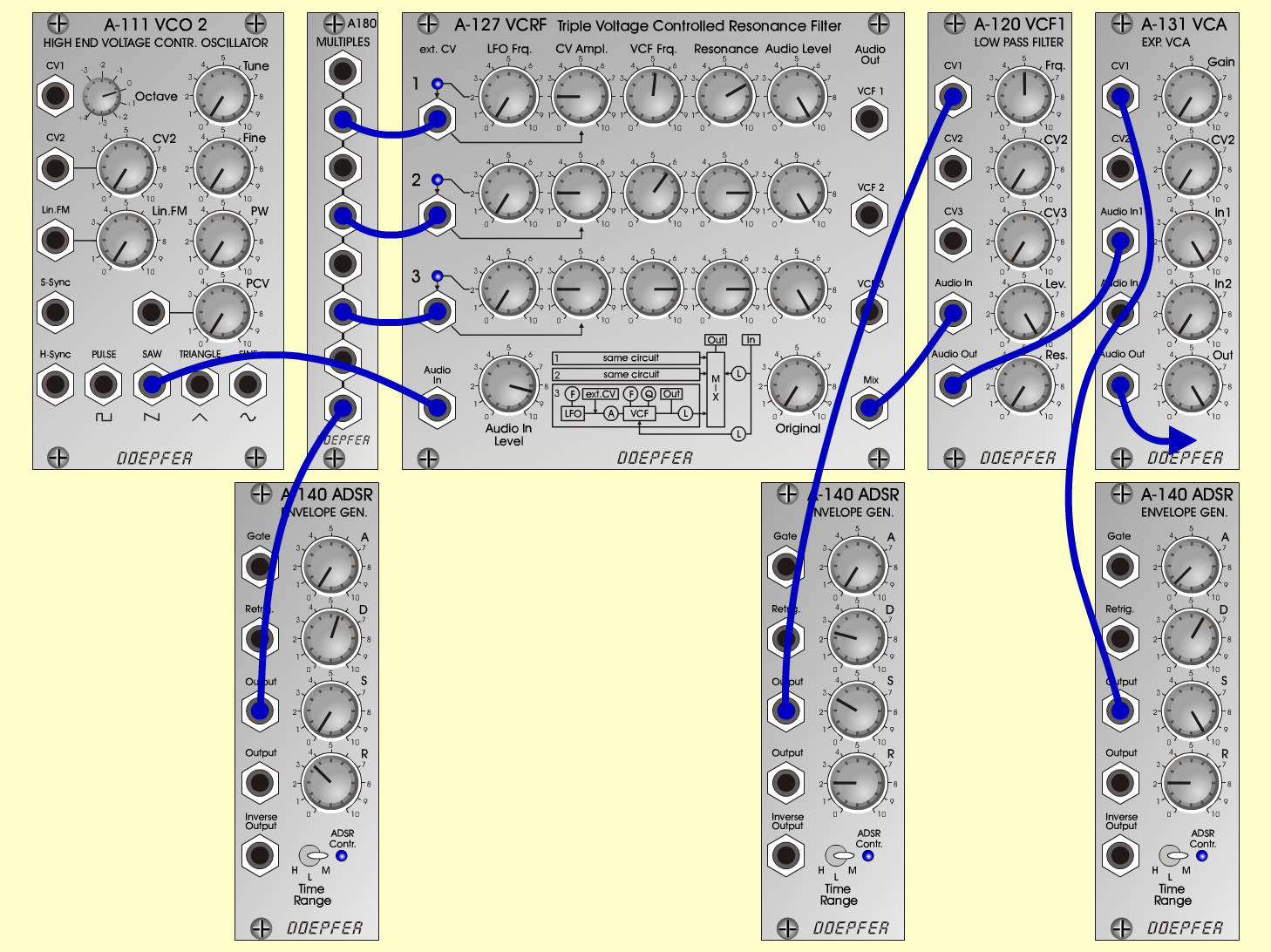JawS y n t hD o e p f e r A - 100 Connections: Settings: A-111 (Saw) <=> A-127 (Audio In)   A-140/1 (Output) <=> A-180   A-180 <=> A-127 (ext. CV 1, 2, 3 In)   A-127 (Mix) <=> A-120 (Audio In)   A-140/2 (Output) <=> A-120 (CV 1)   A-120 (Audio Out) <=> A-131 (Audio In)   A-140/3 (Output) <=> A-131 (CV 1)   A-131 (Audio Out) <=> Amplifier A-127 (Audio In = 8.5, Original = 0; Row 1: CV Ampl = 2, VCF Frq = 5.2, Res = 7, Audio Level = 10; Row 2: CV Ampl = 2, VCF Frq = 6.2, Res = 8, Audio Level = 10; Row 3: CV Ampl = 2, VCF Frq = 8, Res = 8, Audio Level = 10)   A-120 (Freq = 5, Lev = 10, Res = 0)   A-140/1 (A = 0, D = 5.6, S = 0, R = 3.5, Range = M)   A-140/2 (A = 0, D = 2.5, S = 3, R = 2, Range = M)   A-140/3 (A = 0.5, D = 6, S = 10, R = 2, Range = M)   A-131 (Gain = 0, Audio In 1 = 10, Audio Out = 10) Notes: Change A-120 (Freq = 5):   jaw1 - Freq = 3   jaw2 - Freq = 0 Josef MuellerSound samples Mozart: Bona Nox (KV 561) Jaw 1 (130 Hz) Jaw 1 (261 Hz) Jaw 1 (523 Hz) Jaw 2 (130 Hz) Jaw 2 (261 Hz) Jaw 2 (523 Hz)